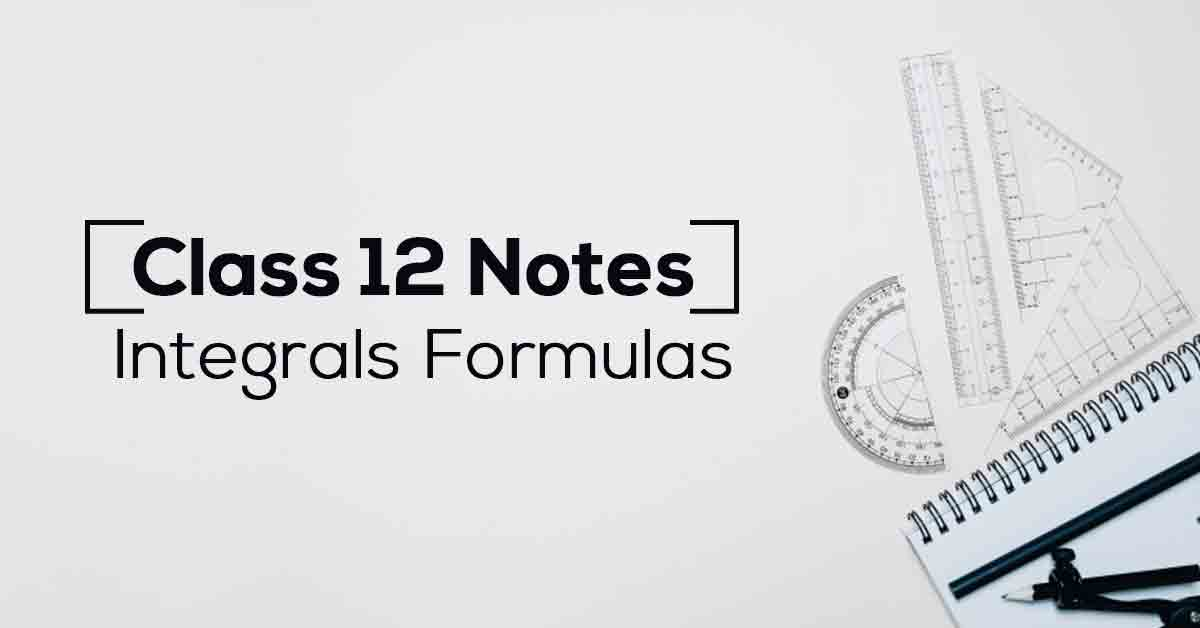Class 12th Math Integrals Formulas CBSE 2023 - Vidyakul

# Integrals Class 12 Formulas PDF with Notes# Chapter 7 Integrals Formulas

Students looking for Class 12 Maths Chapter 7 NCERT notes Integrals can check this article. Students must thoroughly study all the topics to answer the in-text exercise questions. Students can check these notes if they are facing challenges in solving the questions.

Top subject experts prepare these solutions at Vidyakul in accordance with the latest curriculum. Scroll down to get free CBSE Class 7 Maths Chapter 12 notes.

MATHEMATICS NOTES CHAPTER- 7

### Points to Remember

We have provided a few important points that are covered in NCERT Class 12 Maths Chapter 7 Integrals to help students in their exam preparations. Refer to the points below:

• Fundamental Integration formulas:

• ∫ xndx = x(n+1)/(n+1) + C , ∀n ≠ −1

• ∫ x−1dx = ∫ 1/x dx = log |x| + C

• ∫ exdx = ex + C

• ∫ axdx = ax/log a + C

• ∫ logx dx = xlogx − x + C

• First fundamental Theorem of Integral Calculus:

• Let f be a continuous function of x for a ≤ x ≤ b and A(x) = ∫xa f(x) dx, then A′(x) = f(x) for all x in [a, b] and A(a) = 0.

### Topics and Sub-topics

Before getting into the details of NCERT notes for 12th-grade Math's Chapter 7 Integrals, let us have an overview of the list of topics included in this chapter:

 Topic Name Topic Name Integration as an Inverse Process of Differentiation Integration by Parts Methods of Integration Definite Integral Integration by Partial Fractions Some Properties of Definite Integrals